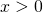# Solution assignment 05 Quadratic inequalities

### Assignment 5

Solve: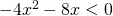### Solution

First we solve the corresponding equation: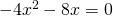or: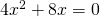The left-hand side can be easily factorized by factoring out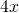: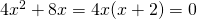and thus the solutions are: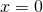or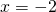The graph of the function in the left-hand side of the inequality is an 'opens down' parabola and thus the following values ofsatisfy the inequality: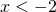or